# JP2013042411A - Image processing apparatus, projector and projector system comprising the image processing apparatus, image processing method, program thereof, and recording medium having the program recorded thereon - Google Patents

Image processing apparatus, projector and projector system comprising the image processing apparatus, image processing method, program thereof, and recording medium having the program recorded thereon Download PDF

## Info

Publication number
JP2013042411A
JP2013042411A JP2011178809A JP2011178809A JP2013042411A JP 2013042411 A JP2013042411 A JP 2013042411A JP 2011178809 A JP2011178809 A JP 2011178809A JP 2011178809 A JP2011178809 A JP 2011178809A JP 2013042411 A JP2013042411 A JP 2013042411A
Authority
JP
Japan
Prior art keywords
image
plane
data
distance
projector
Prior art date
Legal status (The legal status is an assumption and is not a legal conclusion. Google has not performed a legal analysis and makes no representation as to the accuracy of the status listed.)
Granted
Application number
JP2011178809A
Other languages
Japanese (ja)
Other versions
JP5961945B2 (en
Inventor
Shinichi Sumiyoshi

Fumihiro Hasegawa

Original Assignee
Ricoh Co Ltd

Priority date (The priority date is an assumption and is not a legal conclusion. Google has not performed a legal analysis and makes no representation as to the accuracy of the date listed.)
Filing date
Publication date
Application filed by Ricoh Co Ltd, 株式会社リコー filed Critical Ricoh Co Ltd
Priority to JP2011178809A priority Critical patent/JP5961945B2/en
Publication of JP2013042411A publication Critical patent/JP2013042411A/en
Application granted granted Critical
Publication of JP5961945B2 publication Critical patent/JP5961945B2/en
Active legal-status Critical Current
Anticipated expiration legal-status Critical

• 238000003672 processing method Methods 0.000 title claims description 6
• 238000003384 imaging method Methods 0.000 claims abstract description 90
• 230000000875 corresponding Effects 0.000 claims abstract description 83
• 238000004364 calculation methods Methods 0.000 claims abstract description 54
• 238000000926 separation method Methods 0.000 claims abstract description 11
• 239000000284 extracts Substances 0.000 claims description 8
• 238000000034 methods Methods 0.000 description 39
• 238000010586 diagrams Methods 0.000 description 30
• 239000011159 matrix materials Substances 0.000 description 28
• 241001562068 Physidae Species 0.000 description 12
• 238000006243 chemical reactions Methods 0.000 description 12
• 230000001131 transforming Effects 0.000 description 12
• 281000009961 Parallax, Inc. (company) companies 0.000 description 6
• 238000000605 extraction Methods 0.000 description 6
• 230000003287 optical Effects 0.000 description 5
• 238000000611 regression analysis Methods 0.000 description 5
• 101710012747 PGLYRP1 Proteins 0.000 description 4
• 230000004075 alteration Effects 0.000 description 4
• 280000635983 Watermark companies 0.000 description 3
• 239000008264 clouds Substances 0.000 description 3
• 230000005484 gravity Effects 0.000 description 3
• 241000180481 Urva Species 0.000 description 2
• 239000000203 mixtures Substances 0.000 description 2
• 206010010071 Coma Diseases 0.000 description 1
• 241000196252 Ulva Species 0.000 description 1
• 201000009310 astigmatism Diseases 0.000 description 1
• 230000000694 effects Effects 0.000 description 1
• 238000000691 measurement method Methods 0.000 description 1
• 239000010950 nickel Substances 0.000 description 1

## Images

•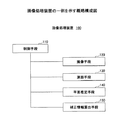•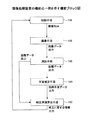•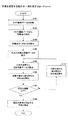•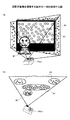•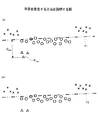•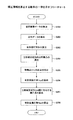•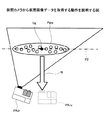•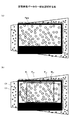•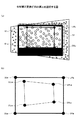•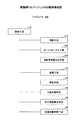•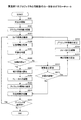•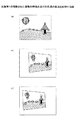•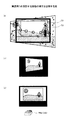••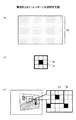•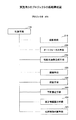•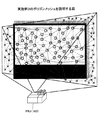••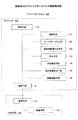•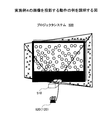## Classifications

• GPHYSICS
• G01MEASURING; TESTING
• G01BMEASURING LENGTH, THICKNESS OR SIMILAR LINEAR DIMENSIONS; MEASURING ANGLES; MEASURING AREAS; MEASURING IRREGULARITIES OF SURFACES OR CONTOURS
• G01B11/00Measuring arrangements characterised by the use of optical means
• G01B11/14Measuring arrangements characterised by the use of optical means for measuring distance or clearance between spaced objects or spaced apertures
• GPHYSICS
• G08SIGNALLING
• G08GTRAFFIC CONTROL SYSTEMS
• G08G1/00Traffic control systems for road vehicles
• G08G1/16Anti-collision systems
• G08G1/168Driving aids for parking, e.g. acoustic or visual feedback on parking space
• HELECTRICITY
• H04ELECTRIC COMMUNICATION TECHNIQUE
• H04NPICTORIAL COMMUNICATION, e.g. TELEVISION
• H04N9/00Details of colour television systems
• H04N9/12Picture reproducers
• H04N9/31Projection devices for colour picture display, e.g. using electronic spatial light modulators [ESLM]
• H04N9/3179Video signal processing therefor
• H04N9/3185Geometric adjustment, e.g. keystone or convergence
• HELECTRICITY
• H04ELECTRIC COMMUNICATION TECHNIQUE
• H04NPICTORIAL COMMUNICATION, e.g. TELEVISION
• H04N9/00Details of colour television systems
• H04N9/12Picture reproducers
• H04N9/31Projection devices for colour picture display, e.g. using electronic spatial light modulators [ESLM]
• H04N9/3191Testing thereof
• H04N9/3194Testing thereof including sensor feedback
• GPHYSICS
• G03PHOTOGRAPHY; CINEMATOGRAPHY; ANALOGOUS TECHNIQUES USING WAVES OTHER THAN OPTICAL WAVES; ELECTROGRAPHY; HOLOGRAPHY
• G03BAPPARATUS OR ARRANGEMENTS FOR TAKING PHOTOGRAPHS OR FOR PROJECTING OR VIEWING THEM; APPARATUS OR ARRANGEMENTS EMPLOYING ANALOGOUS TECHNIQUES USING WAVES OTHER THAN OPTICAL WAVES; ACCESSORIES THEREFOR
• G03B17/00Details of cameras or camera bodies; Accessories therefor
• G03B17/48Details of cameras or camera bodies; Accessories therefor adapted for combination with other photographic or optical apparatus
• G03B17/54Details of cameras or camera bodies; Accessories therefor adapted for combination with other photographic or optical apparatus with projector
• GPHYSICS
• G03PHOTOGRAPHY; CINEMATOGRAPHY; ANALOGOUS TECHNIQUES USING WAVES OTHER THAN OPTICAL WAVES; ELECTROGRAPHY; HOLOGRAPHY
• G03BAPPARATUS OR ARRANGEMENTS FOR TAKING PHOTOGRAPHS OR FOR PROJECTING OR VIEWING THEM; APPARATUS OR ARRANGEMENTS EMPLOYING ANALOGOUS TECHNIQUES USING WAVES OTHER THAN OPTICAL WAVES; ACCESSORIES THEREFOR
• G03B21/00Projectors or projection-type viewers; Accessories therefor
• G03B21/14Details
• G03B21/53Means for automatic focusing, e.g. to compensate thermal effects

## Abstract

PROBLEM TO BE SOLVED: To provide an image processing apparatus capable of calculating information on the correction of an image to be projected, by approximation of an object for projecting the image thereon, by plane.SOLUTION: An image processing apparatus comprises: imaging means which images an area including an object having an image projected thereon, to acquire image data; distance measuring means which calculates distance data on a separation distance between the object and the imaging means on the basis of the image data; plane estimation means which estimates a plane corresponding to the object from the distance data; and correction information calculation means for calculating information on the correction of an image to be projected, on the basis of the distance data and the information on the plane.

## Description

The present invention relates to an image processing apparatus, a projector and a projector system having the image processing apparatus, an image processing method, a program thereof, and a recording medium on which the program is recorded.

A projector is a device that projects an image onto an object such as a screen. When projecting an image, trapezoidal distortion may occur in the projected image depending on the tilt angle between the projector and the object. Some projectors correct (deform) a projected image in advance by an image processing apparatus in order to eliminate trapezoidal distortion of the projected image.

Here, some image processing apparatuses correct an image to be projected based on a separation distance and an inclination angle between a projector and an object.

Patent Document 1 projects a plurality of target points having a predetermined pattern onto a projection target in a planar shape, and based on the distance to the target point, a boundary line between the projection target and the background (of the projection target A technique for detecting a (outer shape) and correcting a projection image (image to be projected) so as to correspond to the boundary line is disclosed.

When projecting an image with a projector, in many cases, the image is projected continuously for a predetermined period. When the object or the projector moves during the image projection, the projector again measures the separation distance and the inclination angle in order to correct the image to be projected.

The technique disclosed in Patent Document 1 can correct the trapezoidal shape of a projected image based on the distance to the object. However, in order to measure the distance, there is a case where the projection of the image by the projector is temporarily stopped and a predetermined pattern or the like is projected again.

The present invention provides an image processing apparatus capable of correcting an image to be projected without interrupting the operation of projecting the image when the relationship between the position of the object and the projector changes during the image projection. The task is to do.

In order to solve the above-described problem, the present invention according to claim 1 is directed to an imaging unit that captures an image including a target object on which an image is projected and acquires image data, and the target based on the image data. A distance measuring unit that calculates distance data regarding a separation distance between an object and the imaging unit, a plane estimation unit that estimates a plane corresponding to the object from the distance data, and the distance data and information about the plane. There is provided an image processing apparatus comprising correction information calculation means for calculating information related to correction of an image to be projected.

According to the present invention, in the image processing apparatus, it is possible to calculate information relating to correction of an image to be projected by estimating a plane corresponding to the object on which the image is projected.

It is a schematic block diagram which shows an example of an image processing apparatus. It is a functional block diagram which shows an example of the function of an image processing apparatus. It is a flowchart which shows an example of the operation | movement which estimates a plane. It is a figure explaining an example of operation which picturizes a projection target object. It is a figure explaining the method to estimate a plane. It is a flowchart which shows an example of the operation | movement which calculates correction information. It is a figure explaining the operation | movement which acquires virtual image data from a virtual camera. It is a figure explaining an example of virtual image data. It is a figure explaining calculation of a trapezoid correction conversion matrix. 1 is a schematic configuration diagram of a projector according to a first embodiment. 6 is a flowchart illustrating an example of a projection operation of the projector according to the first embodiment. FIG. 6 is a diagram illustrating extraction of feature points and corresponding points of a projected image according to the first embodiment. 6 is a diagram illustrating correction of an image to be projected according to the first exemplary embodiment. FIG. FIG. 6 is a schematic configuration diagram of a projector according to a second embodiment. 6 is a diagram illustrating a dot pattern of Example 2. FIG. FIG. 6 is a schematic configuration diagram of a projector according to a third embodiment. It is a figure explaining the polygon mesh of Example 3. FIG. It is a figure explaining the method to estimate a plane from the normal vector of Example 3. FIG. 10 is a schematic configuration diagram of a projector system according to a fourth embodiment. FIG. 10 is a diagram illustrating an example of an operation for projecting an image according to a fourth embodiment.

The present invention will be described in detail using an image processing apparatus that calculates information related to correction of an image to be projected by performing image processing on an image obtained by imaging a region including an object.

(Configuration of image processing apparatus)
FIG. 1 is a schematic configuration diagram illustrating an example of an image processing apparatus.

In FIG. 1, the image processing apparatus 100 includes a control unit 110, an imaging unit 120, a distance measurement unit 130, a plane estimation unit 140, and a correction information calculation unit 150.

The image processing apparatus 100 captures an area including an object on which an image is projected by the imaging unit 120 (hereinafter referred to as a projection object), acquires image data, and the distance measuring unit 130 from the imaging unit 120 to the projection target. Distance data related to the distance to the object is calculated. In addition, the image processing apparatus 100 estimates a plane corresponding to the projection object by the plane estimation unit 140, and corrects the projected image (enlargement / reduction and trapezoid correction) based on the information about the estimated plane and the calculated distance data. And the like, hereinafter referred to as correction).

Here, what can project an image on the external surface, such as a screen, a wall, and a white boat, can be used as the projection object.

The control unit 110 is a unit that controls the entire image processing apparatus 100. The control unit 110 controls the imaging unit 120 and the like based on information input from the outside. The control unit 110 performs control to output information related to the result of image processing of the image processing apparatus 100 based on information input from the outside.

The imaging unit 120 is a unit that forms an image of an area including a projection target on an imaging device (image sensor) and acquires image data related to the image from a pixel output signal (electrical signal) of the imaging device. In this embodiment, the imaging unit 120 includes a stereo camera and a captured image generation unit.

The stereo camera includes two image pickup lenses and two image pickup devices, and simultaneously images a projection target using two image pickup lenses. Here, the imaging lens is for entering an image of the projection object into the imaging device. The imaging element has a light receiving surface in which a plurality of light receiving elements are arranged in a lattice pattern, and forms an image that has passed through the imaging lens and is incident on the light receiving surface.

The captured image generation means generates image data relating to an image of an area including the projection target based on the pixel output signal of the image sensor.

The distance measuring means 130 is a means for measuring the distance from the image processing apparatus 100 (imaging means 120) to the projection target. In the present embodiment, the distance measuring unit 130 calculates the distance from the image processing apparatus 100 (imaging unit 120) to the projection target based on the triangulation principle based on the two image data acquired by the imaging unit 120. . Details will be described later (operation for ranging).

The plane estimation means 140 recursively approximates the plane corresponding to the projection object based on the distance data calculated by the distance measurement means 130. Here, recursively approximating a plane is a method of approximating a plane based on a plurality of positions and then re-estimating the plane by excluding a position away from the estimated plane by a predetermined distance. (Regression analysis method). Details will be described later (operation for estimating a plane).

The correction information calculation unit 150 calculates information related to correction of the projected image based on the information about the plane estimated by the plane estimation unit 140. Details will be described later (operation for calculating correction information).

In the following description, “content image data Aimg” is image data related to an image input to a projection unit (such as a projector) from a PC or the like as data related to the image.

“Camera image data Cimg” is image data relating to an image obtained by imaging the projected content image data Aimg by the imaging unit 120. The camera image data Cimg is generated by digitally processing an electric signal (pixel output signal) such as brightness and darkness of light received by the light receiving element of the imaging unit 120.

“Virtual image data Vimg” is image data related to the camera image data Cimg when it is assumed that the image is taken from the normal direction (hereinafter referred to as the front direction) of the outer surface (projected surface) of the projection target. is there. The virtual image data Vimg is generated using a perspective projection transformation matrix P, which will be described later, based on information about the normal vector calculated by the correction information calculation unit 150.

“Projector image data Pimg” is image data obtained by correcting content image data Aimg in order to eliminate trapezoidal distortion and the like of virtual image data Vimg. The projector image data Pimg is generated using a trapezoidal correction conversion matrix Hpp, which will be described later, based on information related to correction calculated by the correction information calculation unit 150.

(Function of image processing device)
An example of the function of the image processing apparatus will be described with reference to FIG. FIG. 2 is a functional block diagram of the image processing apparatus.

In FIG. 2, the control unit 110 outputs a signal instructing the imaging unit 120 to start imaging in order to start an image processing operation.

The imaging unit 120 captures an image of an area including the projection target with a stereo camera, and acquires two camera image data Cimg. Further, the imaging unit 120 outputs the acquired camera image data Cimg to the distance measuring unit 130.

The distance measuring unit 130 calculates distance data corresponding to a plurality of positions (hereinafter referred to as corresponding points) on the outer surface of the projection target based on the two camera image data Cimg. The distance measuring unit 130 outputs the distance data to the plane estimating unit 140 and the correction information calculating unit 150. Here, the distance data is data relating to the distance from the image processing apparatus to the projection target (corresponding point). Details of the distance measurement method will be described later (operation for distance measurement).

The plane estimation unit 140 calculates regression plane data as a plane corresponding to the projection object from the distance data calculated by the distance measurement unit 130. In addition, the plane estimation unit 140 outputs the regression plane data to the correction information calculation unit 150. Here, the regression plane data is data relating to a plane including a plurality of positions in the three-dimensional space. Details of the estimation method will be described later (operation for estimating a plane).

The correction information calculation unit 150 calculates information related to the correction of the content image data Aimg based on the distance data of the distance measurement unit 130 and the regression plane data of the plane estimation unit 140. Specifically, the correction information calculation unit 150 calculates convex hull data C1 (FIG. 8B), which will be described later, based on the distance data and the regression plane data. Further, the correction information calculation unit 150 cancels (resolves) the trapezoidal distortion and the like of the virtual image data Vimg based on the convex hull data, and the like, a trapezoid correction conversion matrix necessary for correcting the content image data Aimg ( Hereinafter, the correction information is calculated. Details of the calculation method will be described later (operation for calculating correction information).

Further, the correction information calculation means 150 outputs information related to correction to the projection means (not shown) by the control means 110.

(Ranging operation)
The operation in which the distance measuring means calculates distance data related to the distance from the imaging means (image processing apparatus) to the corresponding point (projection object) by the stereo camera of the imaging means will be described.

Here, the stereo camera includes a first camera (reference camera) and a second camera (reference camera), and the first camera and the second camera are a first imaging lens and a second imaging lens. And a first imaging element and a second imaging element which are arranged in the direction of the back side of the first imaging lens and the second imaging lens (direction opposite to the direction of the projection object). As the image sensor, an area sensor, a surface sensor, a two-dimensional sensor, or the like can be used.

The first imaging lens and the second imaging lens are juxtaposed at a predetermined distance D (hereinafter referred to as a baseline length), and the optical axis of the first imaging lens and the optical axis of the second imaging lens are Parallel. In addition, the first image sensor or the like includes a light receiving surface that forms an image of an object on the front surface (opposite the back surface). The optical axis of the first imaging lens or the like is positioned so as to coincide with the center of the diagonal line of the light receiving surface of the first imaging element or the like.

The first image of the projection object that has entered through the first imaging lens and the second image of the projection object that has entered through the second imaging lens are displaced by parallax Δ, respectively. An image is formed on the light receiving surface. The imaging device photoelectrically converts light and darkness of the first image and the second image by the amount of electric charge, and outputs the result as a pixel output signal to the distance measuring unit. At this time, the distance measuring means compares the pixel output signals and detects the parallax Δ from the position (coordinates) of the light receiving element (pixel) on the light receiving surface. Here, assuming that the parallax Δ, the base line length D, the separation distance L between the image processing apparatus and the projection object, and the focal length f of the imaging lens, Equation 1 is established under the condition of L >> f (triangulation) principle).

In this case, D and f are known.

The distance measuring means calculates the separation distance L from Equation 1 using the detected parallax Δ.

Next, the operation in which the distance measuring unit calculates the absolute coordinates (XYZ coordinates) of the corresponding points will be specifically described. Here, the X axis is the direction of the base length D, the Y axis is the direction on the light receiving surface of the image sensor orthogonal to the X axis, the Z axis is the direction orthogonal to the X axis and the Y axis (the optical axis direction of the stereo camera) To do. In addition, the relative coordinates (xyz coordinates) of the first camera (subscript r) and the second camera (subscript l) with respect to the light receiving surface are represented by Formula 2.

At this time, the parallax Δ is expressed by Equation 3.

Next, the coordinate P (absolute coordinate) of the corresponding point is represented by Equation 4.

At this time, the coordinate P of the corresponding point is expressed by Equation 5 from Equations 1 to 3.

As described above, the distance measuring means calculates the three-dimensional coordinates (absolute coordinates) of the corresponding points on the outer surface of the projection object using the stereo camera of the imaging means, and uses the calculated three-dimensional coordinates as the distances to the corresponding points. It can be acquired as data.

(Operation to estimate the plane)
An operation in which the plane estimation unit and the like estimate a plane corresponding to the projection object will be described with reference to FIGS. FIG. 3 is a flowchart showing an example of the operation of estimating the plane by the plane estimation means or the like. FIG. 4 is a diagram illustrating an example of an operation in which the imaging unit images the projection target object. FIG. 5 is a diagram for explaining a method for estimating a plane by regression analysis.

In FIG. 3, the imaging means images a region including the projection target and acquires camera image data Cimg (step S101). Here, the operation of imaging by the imaging means will be specifically described with reference to FIG.

FIG. 4 shows an operation for imaging a projection object. FIG. 4A is an explanatory view of a projection object on which an image is projected as seen from the front. FIG. 4B is an explanatory view of a projection target or the like on which an image is projected as viewed from above. The circles in the figure indicate positions (feature points) on the surface of the projection target (screen) A1. The Δ mark in the figure indicates the position (feature point) of the presenter A2. The x mark in the figure indicates the position (feature point) on the surface of the wall A3 behind the projection object.

In FIG. 4, the presenter A2 stands in the front direction of the projection target A1. Further, the wall A3 is close to the back direction (the direction opposite to the front direction) of the projection target A1. At this time, the imaging unit built in the projector captures an area including the projection target A1 onto which the content image data Aimg is projected by a projector (projection unit) or the like, and acquires camera image data Cimg.

In step S101 in FIG. 3, when the acquisition of the camera image data Cimg is completed, the imaging unit outputs the camera image data Cimg to the distance measuring unit. Thereafter, the process proceeds to step S102.

In step S102, the distance measuring means, based on the camera image data Cimg output from the imaging means, features points of the region including the projection object (◯, Δ, and × in FIGS. 4A and 4B). ). The operation of the distance measuring means to extract the feature points will be specifically described.

The ranging means first selects an arbitrary pixel as a selection point for one of the two camera image data Cimg acquired by the stereo camera (hereinafter referred to as imaging data A). Next, the distance measuring means compares image information (color, brightness, edge strength, etc.) of the selected point and the eight pixels surrounding the selected point from the imaging data A. At this time, if the image information of the selected point is all larger or smaller than the image information of the surrounding eight pixels, the selected point is extracted as a feature point (x A , y A ). In addition, the distance measuring unit extracts a range having a side of 15 pixels around the feature point as a template block A.

When the feature point extraction is completed, the process proceeds to step S103.

Note that the method of extracting feature points is not limited to the above method. In addition to the above method, any method can be used as long as it can extract a characteristic point on the surface of the projection target. Specific examples of feature points will be described in Example 1 and Example 2 described later.

In step S103, the distance measuring means extracts corresponding points based on the feature points extracted in step S102. The operation in which the distance measuring means extracts the corresponding points will be specifically described.

The distance measuring means selects an arbitrary pixel as a selection point (x B , y B ) for the other of the two camera image data Cimg captured by the stereo camera (hereinafter referred to as imaging data B). In addition, the distance measuring unit selects, as the template block B, a range whose sides are 15 pixels square with the selected point as the center. Next, the distance measuring unit calculates the sum of the image information of the template block A and the template block B, and compares the sum of the two. As a comparison method, methods such as SAD and SSD can be used.

Note that SAD (Sum of Absolute Distance) is a method for obtaining the sum of absolute value differences in the comparison of sums. SSD (Squared Sum of Differences) is a method for obtaining the sum of squares of differences.

Next, a selection point (x B , y B ) of the template block B at which the difference of the sum of the image information is the minimum value is selected as a result of the comparison between the template block A and the template block B. At this time, when the difference is equal to or smaller than a predetermined value, the feature point (x A , y A ) of the imaging data A is associated with the selection point (x B , y B ) of the imaging data B, and the feature point (X A , y A ) (selected point (x B , y B )) is extracted as a corresponding point (x AB , y AB ).

Here, the predetermined value can be a distance corresponding to the projection object and the image processing apparatus or a value corresponding to the depth of field. Further, the predetermined value can be a value determined by numerical calculation, experiment, or the like.

The distance measuring means compares all the feature points extracted from the imaging data A with the selected points of the imaging data B as the corresponding points. At this time, the distance measuring means extracts a plurality of corresponding points (hereinafter referred to as a three-dimensional point group).

When the extraction of the corresponding points is completed, the process proceeds to step S104.

In step S104, the distance measuring unit calculates distance data related to the distance of the three-dimensional point group extracted in step S103. Since the operation for calculating the distance data is the same as (the operation for distance measurement), the description is omitted. When the calculation of the distance data is completed, the process proceeds to step S105.

In step S105, the plane estimation unit calculates regression plane data as plane information corresponding to the projection target based on the distance data calculated by the distance measurement unit. A method for calculating the regression plane data will be specifically described with reference to FIG.

FIG. 5A shows the regression plane P1 after the regression analysis. FIG. 5B shows the regression plane P2 after excluding the corresponding point farthest from the plane estimated in step S109 described later.

In FIG. 5A, n corresponding points (X ABi , Y ABi , Z ABi ) (i = 1 to n) are calculated as a three-dimensional point group by steps S102 to S104 (in the drawing). (Circle), (triangle | delta), and x).

Since the plane estimation means calculates the regression plane from the three-dimensional point group by regression analysis, the regression plane equation is defined as z = ax + by + c. At this time, Equation 6 is established between the regression plane and the three-dimensional point group.

Here, the variable of Expression 6 is Expression 7.

In Equation 7, e i indicates a residual.

Next, the normal equation is expressed by Equation 8.

Therefore, β is given by Equation 9.

Thus, using the method of least squares, constants the sum of squares of residuals e i is minimized (parameter) a, b, and by calculating the c, P1 and the regression plane (FIGS. 5 (a) P2) can be determined. The plane estimation means acquires constants a, b, and c of the regression plane equation (z = ax + by + c) as the regression plane data. When the acquisition of the regression plane data is completed, the process proceeds to step S106.

Next, in step S106 of FIG. 3, a distance D ABi between the regression plane and the three-dimensional point group is calculated, and the corresponding point P MAX (X ABD , Y ABD , Z ABD ) of the three-dimensional point group farthest from the regression plane is calculated. ) And its distance D MAX (P MAX in FIG. 5A). Specifically, the distance from the corresponding point (X ABi , Y ABi , Z ABi ) to the plane (αx + βy + γz + δ = 0) is calculated from Equation 10.

The distance between the regression plane and all points in the three-dimensional point group is calculated, and the corresponding point having the maximum absolute value of the distance is selected. When the extraction of the farthest corresponding point P MAX (X ABD , Y ABD , Z ABD ) is completed, the process proceeds to step S107.

In step S107, the distance D MAX relating to the corresponding point P MAX (X ABD , Y ABD , Z ABD ) is compared with a predetermined distance. If the distance D MAX is equal to or smaller than the predetermined distance, the process proceeds to step S108. If the distance D MAX exceeds the predetermined distance, the process proceeds to step S109.

Here, the predetermined distance can be a value corresponding to the separation distance between the projection target and the image processing apparatus, and the distance can be determined by numerical calculation, experiment, or the like. Moreover, it can be set as the value corresponding to the depth of field.

In step S108, the calculated regression plane (step S105) is estimated as a plane corresponding to the projection object, and stored as regression plane data. Thereafter, the process proceeds to “END” in the drawing, and the estimation of the plane is finished.

In step S109, the corresponding point P MAX (X ABD , Y ABD , Z ABD ) is excluded from the three-dimensional point group. When the exclusion is completed, the process returns to step S105, steps S105 to S107 are repeated, and the regression plane P2 is estimated again (FIG. 5B).

As described above, the image processing apparatus of the present invention can measure the distance from the image processing apparatus to the projection object and estimate the plane corresponding to the projection object by regression analysis. Further, the image processing apparatus of the present invention excludes corresponding points whose distance from the plane exceeds a predetermined distance, so that there is an obstacle between the image processing apparatus and the projection object, or the projection object Even when a wall or the like is close to the back of the object, the plane corresponding to the projection object can be estimated.

In addition, in the operation of estimating the plane, the corresponding points to be excluded are not limited to those related to the obstacle and the back wall, but can include things other than the projection target. In addition, the 3D point cloud used when estimating the plane is other than “randomly selected points” and “randomly selected points” from the 3D point cloud extracted in (ranging operation). A constant (parameter) of a plane composed of the point group is calculated, and the plane can be estimated using a point group having a small difference between the constants.

(Operation to calculate correction information)
The operation in which the correction information calculation means calculates the correction information will be described with reference to FIGS.

FIG. 6 is a flowchart illustrating an example of an operation for calculating correction information. FIG. 7 is a diagram illustrating an operation of acquiring virtual image data from a virtual camera. FIG. 8 is a diagram for explaining virtual image data. FIG. 9 is a diagram for explaining the calculation of the trapezoidal correction conversion matrix.

In FIG. 6, the correction information calculation unit calculates image data (virtual image data Vimg) related to the camera image data Cimg when it is assumed that the image is taken from the front direction of the plane estimated by the plane estimation unit (step S201). The operation in which the correction information calculation means calculates the virtual image data Vimg will be specifically described with reference to FIG.

FIG. 7 assumes that a virtual camera (such as a projector including an imaging unit) PRJv is arranged on an extension line in the normal direction N of the plane from the center (center of gravity) position Cg of the three-dimensional point group Pgrp on the estimated plane. Indicates.

In FIG. 7, an actual camera (such as a projector including an imaging unit) PRJr projects content image data Aimg. At this time, the imaging means mounted on the actual camera PRJr acquires the camera image data Cimg, the distance measurement means acquires the distance data of the three-dimensional point group Pgrp, and the plane estimation means estimates the plane P2.

The correction information calculation means calculates virtual image data Vimg captured from the virtual camera PRJv on the extension line in the normal direction N of the plane P2. Specifically, the correction information calculation means projects the three-dimensional point group Pgrp onto a two-dimensional plane (a plane corresponding to the virtual image data Vimg captured from the virtual camera PRJv) using the perspective projection transformation matrix P. Thus, the virtual image data Vimg is calculated.

Here, the perspective projection transformation matrix P is expressed by Equation 11 where A is an internal parameter, R is a rotation matrix that is an external parameter, and t is a translation vector.

Here, the internal parameter A is a matrix (3 × 3) determined by using the optical axis coordinates of the virtual camera PRJv, the scale of the row and column of the image sensor, the focal length f, and the like. The rotation matrix R is a matrix (3 × 3) indicating the rotation from the actual camera PRJr to the virtual camera PRJv. The translation vector t is a vector (3 × 1) indicating translation from the actual camera PRJr to the virtual camera PRJv.

When the calculation of the virtual image data Vimg is completed, the process proceeds to step S202.

Next, in step S202 of FIG. 6, the correction information calculation means calculates convex hull data in the calculated virtual image data Vimg. Here, the convex hull data is data regarding a polygon (hereinafter referred to as a convex hull) that wraps a plurality of corresponding points calculated by the distance measuring means on the plane estimated by the plane estimating means in the three-dimensional point group. is there. A method for calculating the convex hull data will be specifically described with reference to FIG.

FIG. 8 is a diagram illustrating virtual image data Vimg acquired by the virtual camera PRJv. FIG. 8A is a diagram for explaining corresponding points in the virtual image data Vimg. FIG. 8B is a diagram for explaining convex hull data and a trapezoidal correction rectangle described later.

In FIG. 8A, the correction information calculation means includes a plurality of correspondences on the plane estimated by the plane estimation means based on the distance data calculated by the distance measurement means in the virtual image data Vimg acquired by the virtual camera PRJv. A point (◯ in the figure, three-dimensional point group Pgrp in FIG. 7) is extracted. Next, in FIG. 8B, the correction information calculation means calculates convex hull data relating to the convex hull that wraps the corresponding points for the extracted corresponding points. At this time, the convex hull is a polygon C1 including a circle in the figure.

When the calculation of the convex hull data is completed, the process proceeds to step S203.

Next, in step S203 of FIG. 6, the correction information calculation unit calculates a trapezoid correction rectangle in the virtual image data Vimg. Here, the trapezoidal correction rectangle is a rectangle having the maximum area included in the convex hull C1 calculated in step 202. The correction information calculation means calculates the trapezoid correction rectangle C2 included in the convex hull C1 while fixing the aspect ratio (FIG. 8B). The aspect ratio can be the same as the aspect ratio of the image related to the projector image data Pimg. For example, when an image of 1600 × 1200 pixels is projected, the aspect ratio can be 4: 3.

When the calculation of the trapezoidal correction rectangle is completed, the process proceeds to step S204.

In step S204 of FIG. 6, the correction information calculation means selects any four points (M V1 to M V4 in FIG. 8B) in the trapezoidal correction rectangle C2 as feature points. Here, the arbitrary four points can be the four corner points of the projection light related to the virtual image data Vimg. In this case, even when an image with few feature points (or feature points cannot be extracted) is projected, the four corner points can be extracted as feature points. When selection of the feature points is completed, the process proceeds to step S205.

In step S205, the correction information calculation means extracts the corresponding points of the content image data Aimg corresponding to the feature points (M V1 to M V4 ). The operation for extracting the corresponding points is the same as the (ranging operation), and the description is omitted. When the extraction of the corresponding points is completed, the process proceeds to step S206.

In step S206, the correction information calculation means calculates a projective transformation matrix Hcp. Specifically, four corresponding points on the image relating to the content image data Aimg corresponding to the feature point m Vi (x Vi , y Vi ) on the image relating to the virtual image data Vimg are represented by m ai = (x ai , y ai ) (I = 1 to 4), the projective transformation matrix Hcp and Equation 12 hold.

Here, the right side and the left side of Equation 12 are equal in the homogeneous coordinate system (except for a constant multiple of all components). Further, the projective transformation matrix Hcp is the following equation.

At this time, Expression 12 can be expressed as Expression 14.

When the right side is normalized in order to adjust the third component of Equation 14 to 1, Equation 15 is obtained.

Here, h 1 to h 8 are unknown coefficients. By obtaining four combinations of corresponding points M ai (x ai , y ai ) on the image relating to the content image data Aimg corresponding to the feature points M Vi (x Vi , y Vi ) on the image relating to the virtual image data Vimg, h 1 to h 8 can be calculated. As a result, the projective transformation matrix Hcp can be calculated using h 1 to h 8 .

When the calculation of the projective transformation matrix Hcp is completed, the process proceeds to step S207.

In step S207, the correction information calculation means calculates four correction points related to the content image data Aimg corresponding to the four corners of the trapezoid correction rectangle C2 in step S203. A method of calculating the correction point will be specifically described with reference to FIG.

FIG. 9 is a diagram for explaining the relationship between virtual image data Vimg and projector image data Pimg. FIG. 9A is a diagram illustrating the four corner points of the trapezoidal correction rectangle on the image relating to the virtual image data Vimg. FIG. 9B is a diagram for explaining four correction points on the image related to the content image data Aimg.

In FIG. 9A, the four corner points (URv, ULv, DRv, and DLv in the figure) of the trapezoidal correction rectangle C2 calculated in step S203 are extracted. Next, in FIG. 9B, four correction points (URva, ULva, DRva, and DLva in the figure) related to the content image data Aimg corresponding to the four corner points are calculated in step S206. Calculation is performed using the projective transformation matrix Hcp.

When the calculation of the four correction points is completed, the process proceeds to step S208.

In step S208 in FIG. 6, the correction information calculation means converts the four corner points (such as URa in FIG. 9B) of the image relating to the content image data Aimg to the four correction points (such as URva) calculated in step S207. A trapezoidal correction conversion matrix Hpp to be deformed (corrected) is calculated. The method for calculating the trapezoid correction conversion matrix Hpp is the same as that in step S206, and thus the description thereof is omitted.

When the calculation of the trapezoidal correction conversion matrix Hpp is completed, the trapezoidal correction conversion matrix Hpp is stored as information relating to correction, the process proceeds to “END” in the figure, and the operation for calculating the correction information is terminated.

Note that, based on the trapezoidal correction conversion matrix Hpp, the content image data Aimg can be corrected to generate projector image data Pimg in which trapezoidal distortion and the like are canceled (resolved).

As described above, the correction information calculation means can calculate information related to correction necessary for eliminating trapezoidal distortion and the like of the projected image based on distance data, regression plane data, convex hull data, and the like. .

(Program and recording medium recording the program)
The program Pr of the present invention relates to a step of capturing an image including an object on which an image is projected by an image capturing unit to acquire image data, and a separation distance between the image capturing unit and the object based on the image data. Executing a step of calculating distance data, a step of estimating a plane corresponding to the object from the distance data, and a step of calculating information on correction of an image to be projected based on the distance data and information on the plane. .

According to this configuration, an effect equivalent to that of the image processing apparatus of the present invention can be obtained.

Further, the present invention may be a recording medium Md that can be read by a computer in which the program Pr is recorded. As the recording medium Md on which the program Pr is recorded, a computer-readable medium such as a flexible disk, a CD-ROM, and a memory card can be used.

(Example)
An image processing apparatus and an image processing method of the present invention will be described using an embodiment of a projector.

The image processing apparatus and the like of the present invention are not limited to the image processing apparatus and the like used for the projector. Any projector other than a projector can be used as long as it calculates information regarding correction of an image to be projected by performing image processing on an image obtained by imaging an area including an object.

The present invention will be described using the projector according to the first embodiment.

(Projector configuration)
FIG. 10 is an example of a schematic configuration diagram of the projector according to the present embodiment.

10, the projector 200 includes a projection unit 210, an autofocus unit 220, and a projection image generation unit 230 in this embodiment. Further, the projector 200 includes a control unit 110, an imaging unit 120, a distance measurement unit 130, a plane estimation unit 140, a correction information calculation unit 150, and a position movement determination unit 160 as an image processing apparatus.

The projector 200 projects an image on the projection target, images a region including the projection target, and estimates a plane corresponding to the projection target. Further, the projector adjusts the focus of the projection lens based on the estimated plane or the like, and corrects the image to be projected.

Here, the projecting unit 210 is a unit that projects an image onto the projection target from the projection lens of the projector. The autofocus unit 220 is a unit that focuses the projection lens of the projector on the projection target. The projection image generating unit 230 is a unit that generates an image projected by the projector.

The position movement determination unit 160 of the image processing apparatus is a unit that determines whether or not the relationship regarding the position between the projector and the projection target has changed (whether the projector and / or the projection target has moved). The control means 110 and the like of the image processing apparatus are the same as in FIG.

(Operation to calibrate the projection)
An operation in which the projector calibrates an image to be projected will be described with reference to FIGS. FIG. 11 is a flowchart showing an example of the projection operation of the projector of this embodiment. FIG. 12 is a diagram for explaining extraction of feature points and corresponding points of a projected image. FIG. 13 is a diagram for explaining correction of an image to be projected.

In FIG. 11, the control unit of the projector outputs an operation instruction to the projection unit or the like in order to start calibration of the operation of projecting an image by an input from the operation panel or the like (step S301). Thereafter, the process proceeds to step S302.

In step S302, the projecting unit projects the image from the projection lens onto the projection object using the content image data Aimg as the projector image data Pimg. Thereafter, the process proceeds to step S303.

In step S303, the imaging unit captures an area including the projection target and acquires camera image data Cimg. When the acquisition is completed, the process proceeds to step S304.

In step S304, the distance measuring unit calculates the distance from the projector (imaging unit) to the projection target (corresponding point) based on the camera image data Cimg. A method of calculating the distance will be specifically described with reference to FIG.

FIG. 12 shows feature points and corresponding points of an image to be projected. FIG. 12A shows an image related to the content image data Aimg. FIG. 12B shows an image related to the camera image data Cimg acquired by the reference camera of the stereo camera of the imaging unit. FIG. 12C shows an image related to the camera image data Cimg acquired by the reference camera.

In FIG. 12A, image feature points relating to the content image data Aimg are shown (◯ in the figure). The distance measuring means, from the images related to the camera image data Cimg of the standard camera and the reference camera, parallax regarding the corresponding point (◯ in FIG. 12 (b) and FIG. 12 (c)) corresponding to the feature point (FIG. 12 (a)). Δ is calculated. Next, the distance measuring means calculates absolute coordinates (XYZ coordinates) of the corresponding points, and obtains first distance data Dd1. Here, since the method of calculating the absolute coordinates of the corresponding points is the same as the above-described (ranging operation), the description thereof is omitted. When the acquisition of the first distance data Dd1 is completed, the process proceeds to step S305.

In step S305 in FIG. 11, the plane estimation means estimates a plane corresponding to the projection target based on the acquired first distance data Dd1. Here, since the plane estimation method is the same as the above-described (operation for estimating a plane), the description thereof is omitted. When the estimation of the plane is completed, the process proceeds to step S306.

In step S306, the control unit determines whether or not the calibration of the projection operation is the first time (the first calibration after the projector is turned on). If it is the first time, the process proceeds to step S307. Otherwise, the process proceeds to step S312.

In step S307, the correction information calculation unit calculates virtual image data Vimg based on the camera image data Cimg acquired by the imaging unit and the plane estimated by the plane estimation unit. Further, the correction information calculation means calculates information (such as a trapezoid correction conversion matrix Hpp) related to the correction of the projector image data Pimg based on the virtual image data Vimg and the distance data. A method for calculating information related to correction will be specifically described with reference to FIG.

FIG. 13 is a diagram for explaining an operation for correcting an image to be projected. FIG. 13A shows an image related to the virtual image data Vimg before correction. FIG. 13B shows an image related to the corrected projector image data Pimg. FIG. 13C shows an image related to the corrected virtual image data Vimg.

In FIG. 13A, the correction information calculation means generates an image related to the virtual image data Vimg based on the estimated plane of the camera image data Cimg. In addition, the correction information calculation unit calculates the convex hull C1 and the trapezoid correction rectangle C2 based on the image related to the virtual image data Vimg. Next, in FIG. 13B, the correction information calculation means calculates a projective transformation matrix Hcp and a trapezoidal correction conversion matrix Hpp based on the convex hull C1 and the trapezoidal correction rectangle C2. Here, since the method for calculating the projective transformation matrix Hcp and the like is the same as the above-described (operation for calculating correction information), the description thereof is omitted.

When the calculation is completed, the process proceeds to step S308.

In step S308 of FIG. 11, the autofocus unit performs the projection object by moving the projection lens based on the information about the calculated first distance data Dd1 (step S304) and / or the estimated plane (step S305). Adjust the focus of the projection lens. Here, with respect to the focusing method, first distance data Dd1 relating to the distance of the projection object with respect to the position at the center of the projected area is calculated by the distance measuring means, and based on the calculated first distance data Dd1, The focus can be adjusted. The center position may be an average value of points (positions) indicating the maximum and minimum values of the X and Y coordinates of the three-dimensional point group. Or it is good also as an average value (or gravity center) of all the points of a three-dimensional point group.

When focusing is completed, the process proceeds to step S309.

In step S309 in FIG. 11, the projection image generation unit corrects an image related to the projector image data Pimg to be projected. Specifically, the projection image generation unit corrects (deforms) an image related to the content image data Aimg based on the calculated trapezoidal correction conversion matrix Hpp, and an image related to the projector image data Pimg from which the trapezoidal distortion or the like is eliminated. Generate (FIG. 13B). FIG. 13C shows an image related to the virtual image data Vimg when the projector image data Pimg after the correction is projected, and an image in which the trapezoidal distortion is canceled (resolved) when viewed from the front direction of the projection object. Become.

When the correction is completed, the process proceeds to step S310.

In step S310, the control means determines whether or not a predetermined time has passed by using a time counter or the like that measures a predetermined time interval. When a predetermined time elapses, the time counter and the like are reset, and the process proceeds to step S311. If the predetermined time has not elapsed, the system waits until the predetermined time elapses.

In step S311, the control unit determines whether the projector is in use. When the projector is in use, the process returns to step S303 in order to calibrate the operation of projecting at a predetermined time interval. In other cases, the process proceeds to “END” in the figure, and the operation such as calibration is terminated.

In step S312, the position movement determination unit calculates the amount of change in the relationship regarding the position between the projector and the projection target. Specifically, the position movement determination means estimates a new plane by the plane estimation means, and based on a comparison between the previously estimated plane and the newly estimated plane, the positional relationship (separation distance, horizontal angle, elevation angle, etc.) ) Can be calculated. Further, the position movement determination means can set the movement amount of the position of the center of gravity (center) of the trapezoidal correction rectangle based on the estimated plane as the change amount.

When the calculation is completed, the process proceeds to step S313.

In step S313, the position movement determination unit determines whether or not the relationship regarding the position between the projector and the projection target has changed. Specifically, the position movement determination unit can determine that the relationship regarding the position has changed when the amount of change calculated in step S312 exceeds a predetermined amount of change. Here, the predetermined amount of change can be a distance corresponding to the distance between the projector and the projection object or the depth of field. Further, the predetermined change amount can be a value determined by numerical calculation, experiment, or the like.

Thereafter, if it is determined that the relationship regarding the position has changed, the process proceeds to step S314. If it is determined that the positional relationship has not changed, the process proceeds to step S310.

In step S314, the correction information calculation unit calculates information related to correction similar to that in step S307. When the calculation is completed, the process proceeds to step S315.

In step S315, the autofocus unit performs the same focusing as in step S309. When focusing is completed, the process proceeds to step S316.

In step S316, the projection image generation unit corrects the image related to the projector image data Pimg as in step S308. When the correction is completed, the process returns to step S303.

As described above, the projector according to the present embodiment can project an image (projector image data) without interrupting the operation of projecting the image when the relationship between the position of the projector and the projection object changes during projection of the image. Pimg can be corrected. Further, the projector can determine whether or not the relationship regarding the position between the projector and the projection object has changed by comparing the positions of a plurality of planes estimated by the plane estimation means at predetermined time intervals. Furthermore, when the projector determines that the position-related relationship has changed, the projector can correct the projected image without interrupting the operation of projecting the image.

The present invention will be described using the projector of Embodiment 2.

(Projector configuration)
FIG. 14 is an example of a schematic configuration diagram of the projector of the present embodiment.

In FIG. 14, the configuration of the projector 300 is the same as that of the first embodiment (FIG. 10), and thus description thereof is omitted.

(Operation to extract feature points)
An operation in which the distance measuring unit of the projector extracts the feature points will be described. Since the basic operation is the same as the above-described (ranging operation), description thereof is omitted.

In the present embodiment, a pattern image is embedded as an electronic watermark in an image to be projected (image relating to projector image data Pimg), and the pattern image is extracted as a feature point. Here, a dot pattern etc. can be used for a pattern image.

The operation of extracting feature points based on the pattern image will be specifically described with reference to FIG.

FIG. 15 shows an example in which a dot pattern is used as a pattern image. FIG. 15A shows a dot pattern Dp embedded as a digital watermark. FIG. 15B shows a search pattern Sp when extracting feature points. FIG. 15C shows an image in which a dot pattern is embedded.

From FIG. 15C, in the image (camera image data Cimg) projected with the dot pattern Dp (FIG. 15A) embedded, image information (pixel information corresponding to the dot pattern Dp and surrounding pixels) is obtained. The pixel corresponding to the dot pattern Dp becomes an isolated point. In the present embodiment, in FIG. 15B, this isolated point Lp is extracted using the search pattern Sp and set as a feature point. Thereby, when projecting an image with few (or impossible to extract) feature points, the feature points can be extracted by projecting the pattern image (dot pattern) as a digital watermark.

The present invention will be described using the projector of Embodiment 3.

(Projector configuration)
FIG. 16 is an example of a schematic configuration diagram of the projector according to the present embodiment.

In FIG. 16, the configuration of the projector 400 is the same as that of the first and second embodiments, and a description thereof will be omitted.

(Operation to estimate the plane from the normal vector)
An operation in which the plane estimation unit calculates a normal vector using a polygon mesh and estimates a plane corresponding to the projection target based on the normal vector will be described. The basic operation is the same as the above-described (operation for estimating a plane), and thus description thereof is omitted.

In this embodiment, a polygon mesh corresponding to the three-dimensional point group is calculated from the absolute coordinates (second distance data D2) of the three-dimensional point group calculated by the distance measuring means. Here, the polygon mesh is an element that represents an object by a combination of polygons such as triangles. FIG. 17 shows a three-dimensional point group expressed by a polygon mesh. In this embodiment, a three-dimensional point group is expressed by a triangular polygon mesh.

Next, an operation for estimating a plane corresponding to a projection target from a three-dimensional point group expressed by a polygon mesh will be described with reference to FIG.

FIG. 18 shows a normal vector of a polygon mesh calculated based on the absolute coordinates (second distance data D2) of the three-dimensional point group. In this embodiment, a normal vector of each element of the polygon mesh is obtained, a normal vector N obtained by averaging the normal vectors of each element is calculated, and a plane perpendicular to the averaged normal vector N corresponds to the projection object. Presumed to be a flat surface. Further, by comparing the averaged normal vector N with the normal vector of each element, it is possible to detect a projectable range (such as a screen range) of the projection target.

Specifically, correspondence with respect to the normal vectors (D1 and D2 in the figure) of each element in which the difference between the direction of the normal vector of each element and the vector direction of the averaged normal vector N is outside a predetermined range A point is detected, and the position of the detected corresponding point is set as a corresponding point located on the outer shape of the projection target. Thereby, there is no need to perform recursive approximation of (the operation of estimating a plane), and it is possible to calculate the plane and the projectable range (outer shape) of the projection target object by a single calculation.

Also, when the average normal vector is calculated sequentially from the polygon mesh near the center of the captured image, and the difference between the calculated average normal vector and the calculated normal vector exceeds a predetermined value In addition, it can be estimated to be located on the outer shape of the projection object.

Further, the normal vector may be calculated by Expression 16.

In Equation 16, H is a projective transformation matrix in an image captured by the standard camera and reference camera of the stereo camera (imaging means), N b and Ni are internal parameters of the standard camera and reference camera, and R and t are the standard camera and reference. Rotation and translation vectors representing the relative position and orientation of the camera, z is the distance from the stereo camera to the projection object, and N is a normal vector with respect to the estimated reference camera.

The present invention will be described using the projector system of the fourth embodiment.

(Projector system configuration)
FIG. 19 is an example of a schematic configuration diagram of the projector system of the present embodiment.

In FIG. 19, the projector system 500 includes a projector 510 and external means 520. Since the basic configuration of the projector 510 is the same as that of the first to third embodiments, the description thereof is omitted.

The projector 510 includes a communication unit 511 in this embodiment. Similarly, the external unit 520 includes a communication unit 521. The projector 510 and the external unit 520 can communicate with each other via a communication unit 511 and a communication unit 521 in a wired or wireless manner. The external means 520 can use cloud computing or the like.

The external unit 520 includes an imaging unit 120. The external unit 520 can output data related to the image captured by the imaging unit 120 to the projector 510.

(Image capture operation)
In projectors (short focus projectors, close-up projectors, etc.) that are used in close proximity to the projection object, use a wide-angle lens (such as a lens with a wide angle of view or a lens with a short focal length) to project the projected image. Take an image. In this case, it is necessary to correct Seidel aberration (spherical aberration, coma aberration, astigmatism, curvature of field, distortion, etc.) of the captured image.

The projector system 500 of this embodiment is a projector system (imaging means) that does not require correction of Seidel aberration by using the imaging means 120 of the external means 520 in a projector that is arranged and used at a position close to the projection target. ).

Further, the projector system 500 can make the projector 510 smaller, lighter, and simplified by using the imaging unit 120 of the external unit 520.

Further, the external unit 520 can use an imaging unit such as a PC. In the case of making a presentation using the projector 510, a PC or the like used at the time of presentation has an imaging unit. The external unit 520 can be an imaging unit included in a PC or the like used for presentation.

120: Imaging means 130: Distance measuring means 140: Plane estimation means 150: Correction information calculation means 160: Position movement determination means 210: Projection means 230: Projection image generation means 100: Image processing apparatus 200, 300, 400: Projector 500 : Projector system Pr: Program Md: Recording medium Dp: Pattern image (dot pattern)
Dd1: first distance data Dd2: second distance data

JP 2005-229415 A

## Claims (10)

1. Imaging means for capturing an image including a target object on which an image is projected, and acquiring image data;
Ranging means for calculating distance data related to a separation distance between the object and the imaging means based on the image data;
Plane estimation means for estimating a plane corresponding to the object from the distance data;
Correction information calculating means for calculating information relating to correction of an image to be projected based on the distance data and information on the plane;
An image processing apparatus comprising:
2. Position movement determining means for determining whether or not the relationship between the position of the imaging means and the object has changed;
The imaging means captures the area at predetermined time intervals to obtain a plurality of image data,
The ranging means calculates a plurality of first distance data corresponding to the predetermined time interval from the plurality of image data,
The plane estimation means estimates a plurality of planes from the first distance data,
In the plurality of planes, the position movement determination unit compares a plane corresponding to the predetermined time interval with another plane, thereby changing a relationship regarding a position between the imaging unit and the object. Whether or not
The correction information calculation means calculates information related to the correction based on the relation regarding the position after the change when the position movement determination means determines that the relation regarding the position has changed.
The image processing apparatus according to claim 1.
3. The projected image includes a pattern image,
The image processing apparatus according to claim 1, wherein the distance measuring unit extracts the pattern image and calculates distance data corresponding to the pattern image.
4. The distance measuring means calculates a plurality of second distance data corresponding to the area,
The plane estimation means calculates a plurality of normal vectors based on the second distance data, and estimates the plane from the normal vectors.
The image processing apparatus according to claim 1, wherein the image processing apparatus is an image processing apparatus.
5. An image processing apparatus according to any one of claims 1 to 4,
A projection image generating means for correcting an image to be projected based on the information relating to the correction;
Projecting means for projecting the projected image corrected by the projection image generating means;
Having
A projector characterized by that.
6.   The projector system having a projector according to claim 5, wherein the projection image generation unit communicates with the projector by wireless or wired communication.
7. Imaging an area including an object onto which an image is projected by an imaging means to obtain image data;
Calculating distance data related to a separation distance between the imaging unit and the object based on the image data;
Estimating a plane corresponding to the object from the distance data;
Calculating information on correction of an image to be projected based on the distance data and information on the plane;
An image processing method comprising:
8. The step of acquiring the image data includes a step of capturing a plurality of image data by imaging the region at a predetermined time interval,
The step of calculating the distance data includes a step of calculating a plurality of first distance data corresponding to the predetermined time interval from the plurality of image data,
Estimating the plane includes estimating a plurality of planes corresponding to the predetermined time interval from the first distance data;
In the plurality of planes, comparing one plane corresponding to the predetermined time interval with another plane to determine whether or not the relationship regarding the position between the imaging unit and the object has changed. When,
Calculating the information regarding the correction based on the relationship regarding the position after the change when determining that the relationship regarding the position has changed in the determining step;
The image processing method according to claim 7, further comprising:
9.   A program for causing a computer to execute the image processing method according to claim 7 or 8.
10.   A computer-readable recording medium on which the program according to claim 9 is recorded.
JP2011178809A 2011-08-18 2011-08-18 Image processing apparatus, projector and projector system having the image processing apparatus, image processing method, program thereof, and recording medium recording the program Active JP5961945B2 (en)

## Priority Applications (1)

Application Number Priority Date Filing Date Title
JP2011178809A JP5961945B2 (en) 2011-08-18 2011-08-18 Image processing apparatus, projector and projector system having the image processing apparatus, image processing method, program thereof, and recording medium recording the program

## Applications Claiming Priority (6)

Application Number Priority Date Filing Date Title
JP2011178809A JP5961945B2 (en) 2011-08-18 2011-08-18 Image processing apparatus, projector and projector system having the image processing apparatus, image processing method, program thereof, and recording medium recording the program
EP20120823869 EP2745504A4 (en) 2011-08-18 2012-08-09 Image processing apparatus, projector and projector system including image processing apparatus, image processing method
PCT/JP2012/070784 WO2013024882A1 (en) 2011-08-18 2012-08-09 Image processing apparatus, projector and projector system including image processing apparatus, image processing method
AU2012295789A AU2012295789B2 (en) 2011-08-18 2012-08-09 Image processing apparatus, projector and projector system including image processing apparatus, image processing method
CN201280039743.9A CN103765870B (en) 2011-08-18 2012-08-09 Image processing apparatus, projector and projector system including image processing apparatus, image processing method
US14/238,689 US9759548B2 (en) 2011-08-18 2012-08-09 Image processing apparatus, projector and projector system including image processing apparatus, image processing method

## Publications (2)

Publication Number Publication Date
JP2013042411A true JP2013042411A (en) 2013-02-28
JP5961945B2 JP5961945B2 (en) 2016-08-03

# Family

## Family Applications (1)

Application Number Title Priority Date Filing Date
JP2011178809A Active JP5961945B2 (en) 2011-08-18 2011-08-18 Image processing apparatus, projector and projector system having the image processing apparatus, image processing method, program thereof, and recording medium recording the program

## Country Status (6)

US (1) US9759548B2 (en)
EP (1) EP2745504A4 (en)
JP (1) JP5961945B2 (en)
CN (1) CN103765870B (en)
AU (1) AU2012295789B2 (en)
WO (1) WO2013024882A1 (en)

## Cited By (10)

* Cited by examiner, † Cited by third party
Publication number Priority date Publication date Assignee Title
JP2014179698A (en) * 2013-03-13 2014-09-25 Ricoh Co Ltd Projector and control method of projector, and program of control method and recording medium with program recorded thereon
JP2014187515A (en) * 2013-03-22 2014-10-02 Seiko Epson Corp Projector and control method therefor
JP2015057876A (en) * 2013-08-13 2015-03-26 株式会社リコー Image processor, image processing system, image processing method, and program
EP2876876A2 (en) 2013-11-22 2015-05-27 Ricoh Company, Ltd. Image projection apparatus, image projection method, and computer-readable storage medium
JP2015139087A (en) * 2014-01-22 2015-07-30 株式会社リコー Projection device
JP2015173431A (en) * 2014-02-18 2015-10-01 パナソニック インテレクチュアル プロパティ コーポレーション オブアメリカＰａｎａｓｏｎｉｃ Ｉｎｔｅｌｌｅｃｔｕａｌ Ｐｒｏｐｅｒｔｙ Ｃｏｒｐｏｒａｔｉｏｎ ｏｆ Ａｍｅｒｉｃａ Projection system and semiconductor integrated circuit
JP2016014712A (en) * 2014-07-01 2016-01-28 キヤノン株式会社 Shading correction value calculation device and shading correction value calculation method
US9560327B2 (en) 2014-02-19 2017-01-31 Ricoh Company, Limited Projection system and projection method
JP2017073705A (en) * 2015-10-08 2017-04-13 富士通株式会社 Projection device, video projection method and computer program for video projection
WO2020013021A1 (en) * 2018-07-13 2020-01-16 株式会社ニコン Detecting device, processing device, detecting method, and processing program

## Families Citing this family (8)

* Cited by examiner, † Cited by third party
Publication number Priority date Publication date Assignee Title
US9105103B2 (en) * 2013-01-23 2015-08-11 Leap Motion, Inc. Systems and methods of tracking object movements in three-dimensional space
KR20150058660A (en) * 2013-11-19 2015-05-29 삼성전자주식회사 Image processing device, method thereof, and system including the same
CN104460197A (en) * 2014-11-25 2015-03-25 深圳先进技术研究院 Projector capable of automatically correcting geometric distortion and correcting method thereof
CN104486573B (en) * 2014-12-22 2017-11-28 联想(北京)有限公司 A kind of information processing method and electronic equipment
DE102015210879A1 (en) * 2015-06-15 2016-12-15 BSH Hausgeräte GmbH Device for supporting a user in a household
US10444006B2 (en) * 2015-08-19 2019-10-15 Faro Technologies, Inc. Three-dimensional imager
US10455216B2 (en) 2015-08-19 2019-10-22 Faro Technologies, Inc. Three-dimensional imager
EP3346699A4 (en) 2015-09-01 2019-08-14 NEC Platforms, Ltd. Projection device, projection method and program storage medium

## Citations (8)

* Cited by examiner, † Cited by third party
Publication number Priority date Publication date Assignee Title
JP2004069996A (en) * 2002-08-06 2004-03-04 Seiko Epson Corp Projector system, and information processor and projector
JP2004088169A (en) * 2002-08-22 2004-03-18 Nisca Corp Image display
JP2005092820A (en) * 2003-09-19 2005-04-07 Sony Corp Environment recognition apparatus and method, path planning apparatus and method, and robot apparatus
JP2006197443A (en) * 2005-01-17 2006-07-27 Seiko Epson Corp Image processing system, projector, program, information recording medium and method for processing image
JP2006304100A (en) * 2005-04-22 2006-11-02 Olympus Corp Multi-projection system and monitoring method
JP2007094036A (en) * 2005-09-29 2007-04-12 Casio Comput Co Ltd Projector, projecting method, and program
JP2010050540A (en) * 2008-08-19 2010-03-04 Seiko Epson Corp Projection display apparatus, and display method
JP2011133273A (en) * 2009-12-22 2011-07-07 Canon Inc Estimation apparatus and control method thereof, and program

## Family Cites Families (3)

* Cited by examiner, † Cited by third party
Publication number Priority date Publication date Assignee Title
JP3880582B2 (en) * 2004-02-13 2007-02-14 Ｎｅｃビューテクノロジー株式会社 Projector with multiple cameras
JP5994301B2 (en) 2011-06-20 2016-09-21 株式会社リコー Image processing apparatus, information processing apparatus, method, program, and recording medium
JP2013033206A (en) 2011-07-06 2013-02-14 Ricoh Co Ltd Projection display device, information processing device, projection display system, and program

## Patent Citations (8)

* Cited by examiner, † Cited by third party
Publication number Priority date Publication date Assignee Title
JP2004069996A (en) * 2002-08-06 2004-03-04 Seiko Epson Corp Projector system, and information processor and projector
JP2004088169A (en) * 2002-08-22 2004-03-18 Nisca Corp Image display
JP2005092820A (en) * 2003-09-19 2005-04-07 Sony Corp Environment recognition apparatus and method, path planning apparatus and method, and robot apparatus
JP2006197443A (en) * 2005-01-17 2006-07-27 Seiko Epson Corp Image processing system, projector, program, information recording medium and method for processing image
JP2006304100A (en) * 2005-04-22 2006-11-02 Olympus Corp Multi-projection system and monitoring method
JP2007094036A (en) * 2005-09-29 2007-04-12 Casio Comput Co Ltd Projector, projecting method, and program
JP2010050540A (en) * 2008-08-19 2010-03-04 Seiko Epson Corp Projection display apparatus, and display method
JP2011133273A (en) * 2009-12-22 2011-07-07 Canon Inc Estimation apparatus and control method thereof, and program

## Cited By (13)

* Cited by examiner, † Cited by third party
Publication number Priority date Publication date Assignee Title
JP2014179698A (en) * 2013-03-13 2014-09-25 Ricoh Co Ltd Projector and control method of projector, and program of control method and recording medium with program recorded thereon
JP2014187515A (en) * 2013-03-22 2014-10-02 Seiko Epson Corp Projector and control method therefor
JP2015057876A (en) * 2013-08-13 2015-03-26 株式会社リコー Image processor, image processing system, image processing method, and program
US9787960B2 (en) 2013-08-13 2017-10-10 Ricoh Company, Limited Image processing apparatus, image processing system, image processing method, and computer program
EP2876876A2 (en) 2013-11-22 2015-05-27 Ricoh Company, Ltd. Image projection apparatus, image projection method, and computer-readable storage medium
EP2876876A3 (en) * 2013-11-22 2015-07-22 Ricoh Company, Ltd. Image projection apparatus, image projection method, and computer-readable storage medium
US9621820B2 (en) 2013-11-22 2017-04-11 Ricoh Company, Limited Image projection apparatus, image projection method, and computer-readable storage medium
JP2015139087A (en) * 2014-01-22 2015-07-30 株式会社リコー Projection device
JP2015173431A (en) * 2014-02-18 2015-10-01 パナソニック インテレクチュアル プロパティ コーポレーション オブアメリカＰａｎａｓｏｎｉｃ Ｉｎｔｅｌｌｅｃｔｕａｌ Ｐｒｏｐｅｒｔｙ Ｃｏｒｐｏｒａｔｉｏｎ ｏｆ Ａｍｅｒｉｃａ Projection system and semiconductor integrated circuit
US9560327B2 (en) 2014-02-19 2017-01-31 Ricoh Company, Limited Projection system and projection method
JP2016014712A (en) * 2014-07-01 2016-01-28 キヤノン株式会社 Shading correction value calculation device and shading correction value calculation method
JP2017073705A (en) * 2015-10-08 2017-04-13 富士通株式会社 Projection device, video projection method and computer program for video projection
WO2020013021A1 (en) * 2018-07-13 2020-01-16 株式会社ニコン Detecting device, processing device, detecting method, and processing program

## Also Published As

Publication number Publication date
EP2745504A4 (en) 2014-09-10
CN103765870A (en) 2014-04-30
US9759548B2 (en) 2017-09-12
AU2012295789B2 (en) 2015-08-13
WO2013024882A1 (en) 2013-02-21
EP2745504A1 (en) 2014-06-25
US20140204204A1 (en) 2014-07-24
CN103765870B (en) 2017-04-12
AU2012295789A1 (en) 2014-02-13
JP5961945B2 (en) 2016-08-03

## Similar Documents

Publication Publication Date Title
US10225543B2 (en) System and methods for calibration of an array camera
CN107025635B (en) Depth-of-field-based image saturation processing method and device and electronic device
US10070120B2 (en) Optical see-through display calibration
CN108463740B (en) Depth mapping using structured light and time of flight
TWI547828B (en) Calibration of sensors and projector
Herrera et al. Accurate and practical calibration of a depth and color camera pair
Raposo et al. Fast and accurate calibration of a kinect sensor
KR101590530B1 (en) Automatic scene calibration
CN102572505B (en) System and method for calibrating a depth imaging sensor
US10288418B2 (en) Information processing apparatus, information processing method, and storage medium
JP2017531976A (en) System and method for dynamically calibrating an array camera
US9344695B2 (en) Automatic projection image correction system, automatic projection image correction method, and non-transitory storage medium
EP2571257B1 (en) Projector device and operation detecting method
KR101259835B1 (en) Apparatus and method for generating depth information
US10059002B2 (en) Image processing apparatus, image processing method, and non-transitory computer-readable medium
JP5018980B2 (en) Imaging apparatus, length measurement method, and program
CN100442141C (en) Image projection method and device
JP4852591B2 (en) Stereoscopic image processing apparatus, method, recording medium, and stereoscopic imaging apparatus
JP5902297B2 (en) Method and apparatus for calibrating an imaging device
US9866818B2 (en) Image processing apparatus and method, image processing system and program
US8384911B2 (en) Measurement device and measurement method
JP6338595B2 (en) Mobile device based text detection and tracking
JP6456156B2 (en) Normal line information generating apparatus, imaging apparatus, normal line information generating method, and normal line information generating program
TWI253006B (en) Image processing system, projector, information storage medium, and image processing method
US7554575B2 (en) Fast imaging system calibration

## Legal Events

Date Code Title Description
A621 Written request for application examination

Free format text: JAPANESE INTERMEDIATE CODE: A621

Effective date: 20140711

A131 Notification of reasons for refusal

Free format text: JAPANESE INTERMEDIATE CODE: A131

Effective date: 20151027

A521 Written amendment

Free format text: JAPANESE INTERMEDIATE CODE: A523

Effective date: 20151217

TRDD Decision of grant or rejection written
A01 Written decision to grant a patent or to grant a registration (utility model)

Free format text: JAPANESE INTERMEDIATE CODE: A01

Effective date: 20160531

A61 First payment of annual fees (during grant procedure)

Free format text: JAPANESE INTERMEDIATE CODE: A61

Effective date: 20160613

R151 Written notification of patent or utility model registration

Ref document number: 5961945

Country of ref document: JP

Free format text: JAPANESE INTERMEDIATE CODE: R151